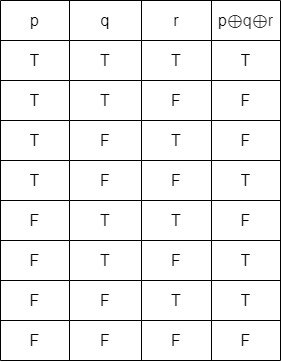# Mathematics - Discrete Mathematics - Implication and Equivalence Statements

in STEMGeeks6 months ago[Image 1]

## Introduction

Hey it's a me again @drifter1!

Today we continue with Mathematics, and more specifically the branch of "Discrete Mathematics", in order to get into more on Implication and Equivalence Statements.

Of course, you should check out the previous part before getting into this one...

So, without further ado, let's dive straight into it!

## Truth Tables

In the previous article, a proposition was defined as a declarative sentence that is either true or false. So, even in compound propositions (which are the combination of multiple propositions) the only thing that matters is the final truth value.

The truth value depends on the chosen truth value of each propositional variable that makes up the statement. There are only two possibilities for each such variable. This allows for a visual representation of all possible combinations via something known as a truth table.

### Logical Connectives

The truth tables of the main logical connectives are the following: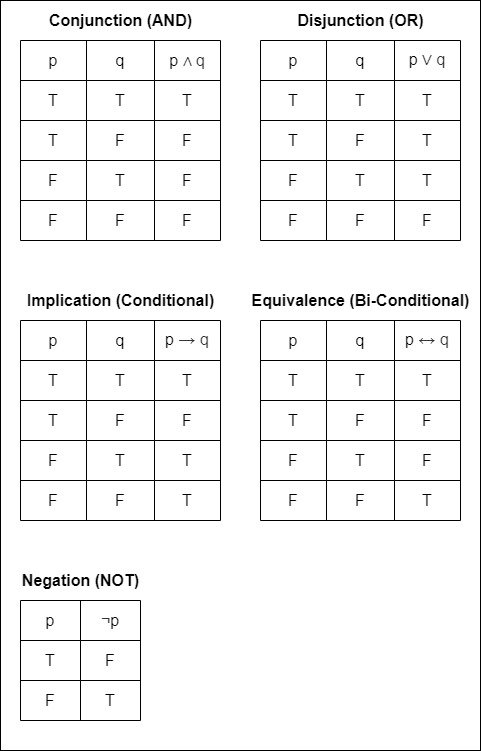where T means true and F means false.

In the context of computer science and mainly logic gates, truth tables are mostly in 0-1 form, where 0 means false and 1 means true.

It's easy to generalize conjunction and disjunction for any number of propositions (inputs):

• the conjunction will have a T only in the row where all propositions are T
• the disjunction will have a F only in the row where all propositions are F

### Exercise

What's the truth table of an Exclusive-OR (XOR) with 3 inputs?

Hint: In the case of two inputs the output is true only when the propositions are not equal. For any number of inputs it is generalized into being true only if the number of true inputs is odd.

The inverse of the XOR is known as a XNOR. It is true when the two inputs are equal, and generally the number of true inputs is even.

## Implication (or Conditional)

An implication is a statement of the form p → q, where p is the hypothesis (or antecedent) and q the conclusion (or consequent). Such mathematical statements can be proven directly by assuming p (p is true) and deducing q from it.

Implications basically specify the relationship between two propositions. If p → q is a proposition then:

• q → p is the converse, which is NOT logically equivalent and a completely independent implication
• ¬q → ¬p is the contrapositive, which IS logically equivalent to the original implication
• ¬p → ¬q is the inverse

## Equivalence (or Bi-Conditional)

If both p → q and q → p are true then p ↔ q. Such a statement is called a bi-conditional statement or an equivalence.

Proving logical equivalence is as simple as proving that two statements have the same truth table or that each can be deduced from the other (proving p → q and q → p respectively).

Logical equivalence is commonly denoted using the symbol, which basically means equivalence.

### Propositional Algebra

Based on logical equivalence it's possible to simplify statements using various laws of algebra. These are: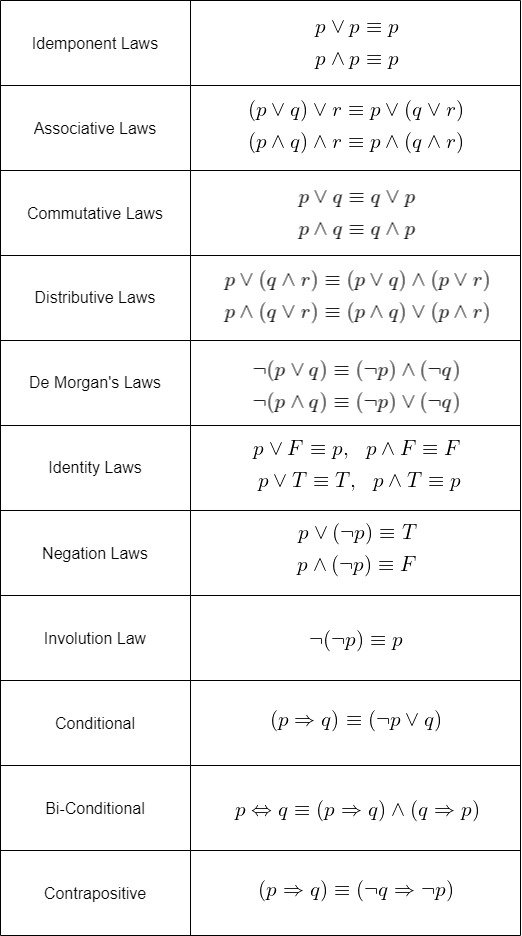### Example

Let's proof the following equivalence: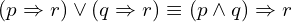For the left-hand side, replacing the conditionals using the respective laws, and simplifying, yields: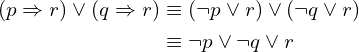For the right-hand side now, replacing the conditional and then applying De Morgan's law yields: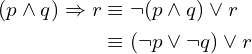So, both result in the same statement, and are thus logically equivalent!

## RESOURCES:

### Images

Mathematical equations used in this article, have been generated using quicklatex.

Block diagrams and other visualizations were made using draw.io.

## Previous articles of the series

• Introduction → Discrete Mathematics, Why Discrete Math, Series Outline
• Sets → Set Theory, Sets (Representation, Common Notations, Cardinality, Types)
• Set Operations → Venn Diagrams, Set Operations, Properties and Laws
• Sets and Relations → Cartesian Product of Sets, Relation and Function Terminology (Domain, Co-Domain and Range, Types and Properties)
• Relation Closures → Relation Closures (Reflexive, Symmetric, Transitive), Full-On Example
• Equivalence Relations → Equivalence Relations (Properties, Equivalent Elements, Equivalence Classes, Partitions)
• Partial Order Relations and Sets → Partial Order Relations, POSET (Elements, Max-Min, Upper-Lower Bounds), Hasse Diagrams, Total Order Relations, Lattices
• Combinatorial Principles → Combinatorics, Basic Counting Principles (Additive, Multiplicative), Inclusion-Exclusion Principle (PIE)
• Combinations and Permutations → Factorial, Binomial Coefficient, Combination and Permutation (with / out repetition)
• Combinatorics Topics → Pigeonhole Principle, Pascal's Triangle and Binomial Theorem, Counting Derangements
• Propositions and Connectives → Propositional Logic, Propositions, Connectives (∧, ∨, →, ↔ and ¬)

## Final words | Next up

And this is actually it for today's post!

Next time we will continue on with strategies for proving mathematical statements...

See ya!Keep on drifting!

Posted with STEMGeeks

Sort:

Awesome post! !1UP#### You have received a 1UP from @mango-juice!

The following @oneup-cartel family members will soon upvote your post:
`@stem-curator`
And they will bring !PIZZA 🍕

Learn more about our delegation service to earn daily rewards. Join the family on Discord.

Solution to Exercise:

A 3-input XOR has the following truth table: